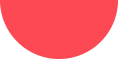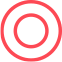## Statistical Data Analysis### Statistical Data Analysis

In order to do statistical analysis, you have to connect the data you have with the insights you need. Using a range of software tools, our statistical consulting team can offer you specialized statistical advice and data analysis with various statistical softwares like SPSS, Stata, R & R studio, EViews, Python, SPSS AMOS, SmartPLS, WarpPLS, JMP etc.

Our statistical consulting team is able to choose the most suitable statistical methods to address your research questions. In terms of statistical analysis, we are well-versed in a wide range of methods, including but not limited to:

• Regression Analysis (linear, multiple, logistic, ordered, multinomial, negative binomial, poisson, probit, logit, tobit, ordered probit & zero-inflated ordered probit etc.)
• Systematic Review & Meta Analysis
• Correlation Analysis & Chi-square testing
• Cluster Analysis & Hierarchical linear modeling (HLM)
• Survival Analysis and Event History Analysis (Kaplan-Meier, Cox regression model, Cox proportional hazard model)
• Time Series Analysis and Panel Data Analysis
• Reliability Analysis, Principal Component Analysis (PCA), Factor Analysis, Discriminant Analysis
• Structural Equation Modeling (SEM), Confirmatory and Exploratory Factor Analysis, Multidimensional Scaling and Path Analysis
• Conjoint Analysis, Choice Modeling/Discrete Choice & Maximum Difference Scaling
• Power Analysis and Sample Sizes
• Hypothesis testing (t‑tests,z-test, ANOVA, MANOVA, ANCOVA, MANCOVA)
• Non-parametric testing (Mann-Whitney U test, Wilcoxon-signed ranked test, Kruskal-Wallis test, Spearman correlation)
• Machine Learning Models (k-means clustering, Hierarchical clustering, K-Nearest Neighbors (KNN), Naïve Bayes Classifier, Classification Tree, Decision Tree, Random Forest, Support Vector Machine (SVM), Artificial Neural Network (ANN))
• Difference-in-Difference (DID), Instrumental Variables (IV), Propensity Score Matching (PSM) and Regression Discontinuity Design (RDD)